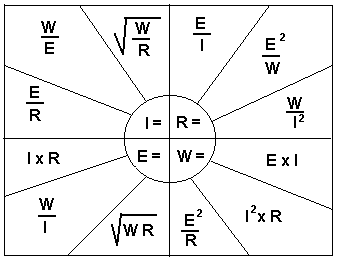Ohms Law ChartThis handy chart shows the forumlas for calculating the Current, Resistance, Voltage, and Watts using ohms law. To use the chart, select the unit to be calculated, then pick one of the three formulas that corresponds to the two known values.
Example 1: To figure Current (I) when Voltage (E) and Resistance (R) are known, the formula would be I = E/R.
Example 2: To figure Wattage (W) when Current (I) and Resistance (R) are known, the formula would be W = I² × R.